# Change Nickname

Current Nickname:

Certifications

Certificate Issued at Valid until Serial No. File
Serial No. Valid until

Not having one? Apply now! >>>

Invoice

ProductName CreateTime ID Price File
ProductName CreateTime ID Price File

# HyperANF

## Overview

Graph average distance is defined as the average length of the shortest paths of all node pairs, it can be used to describe the compactness of the graph. In the early days, this concept was used to evaluate the design of building floors and study the structure of chemical molecular and so on. Gradually, it is applied to the analysis and design of computer system and communication network.

The theoretical value of the graph average distance can be solved by the Neighborhood Function. Since the neighborhood function is very resource intensive when running on large graphs, ANF (Approximate Neighborhood Function) algorithm and HyperANF algorithm - a breakthrough improvement over ANF in terms of speed and linear scalability - were emerged. HyperANF was proposed in 2011 by Paolo Boldi, Marco Rosa and Sebastiano Vigna of the Department of Information Science at the University of Milan, Italy.

Related materials of the algorithm:

## Basic Concept

### Graph Average Distance

According to the definition of graph average distance, given `i` and `j` two distinct nodes in the same connected component, `d(i,j)` is the distance (the length or the number of edges of the shortest path) between `i` and `j`, `k` is the number of all connectable node pairs in the whole graph, then the graph average distance can be expressed as: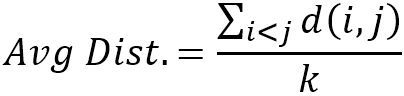Another expression of the graph average distance is given by counting the values of `d(i,j)` in the above formula, by using a positive integer `t` to represent the value of `d(i,j)` and `p(t)` to represent the number of node pairs whose distance is `t`: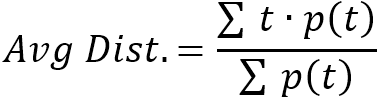As the following figure shows, the two methods to calculate graph average distance are: `(1+2+1+1)/4=1.25` and `(1*3+2*1)/4=1.25`.### Neighborhood Function

Neighborhood Function, also known as Global Neighborhood Function, is denoted as `N(t)` which means when the distance `t` is given, the number of node pairs that have distance of `t` or less; the `p(t)` mentioned earlier can be written by neighborhood function as `p(t) = N(t) - N(t-1)`.

Consider the number of nodes that are equal or less than `t` far from node `x` (those nodes are not `x`), define it as Independent Neighborhood Function and denote as `Nx(t)`; the relationship between independent neighborhood function and global neighborhood function is: `N(t) = 1/2 · ∑ Nx(t)`.Calculate `N(2) = (2+3+4+3+2)/2 = 7` by `Nx(2)`, or calculate `N(2) = p(2) + N(1) = 3 + 4 = 7` by `p(2)`, the results are the same.

So far, the solution of the graph average distance can be converted into the calculation of `Nx(t)`.

### Neighborhood Set

If uses set `B(x,t)` to represent all the nodes that node `x` can reach within `t` steps (`x` included) and call it the `t`-step Neighborhood Set of `x`, then `Nx(t) = |B(x,t)| - 1`; `B(x,t)` can be obtained by the iterative computation of itself, i.e. `B(x,t) = ∪ B(y,t-1)`, where `y` is the 1-step neighbor of `x`.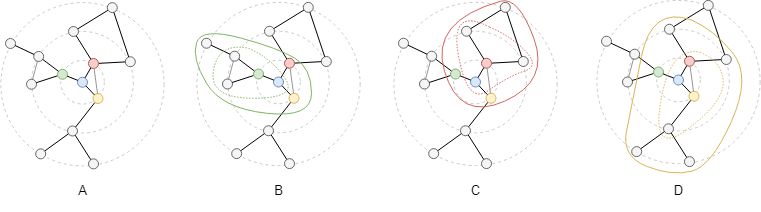To examine the neighborhood set `B(x,3)` of the blue node `x` in Graph A, 1-step neighbors of the blue node are the green, red and yellow three nodes (denoted as `i`, `j`, `k` respectively); the nodes in the green, red and yellow circles in graph B, C, D are `B(i,2)`, `B(j,2)`, `B(k,2)` respectively, the union of the three circles is the whole graph, i.e. `B(x,3) = B(i,2) ∪ B(j,2) ∪ B(k,2)`.

According to the definition of neighborhood set, the calculation of `Nx(t)` is essentially a process of finding the union set and getting the size of the set, that is, writing the elements in multiple sets into a sequence and then finding the cardinality of the sequence. Cardinality refers to the number of distinct elements in a sequence. For example, two sets `{1, 3, 4}` and `{4, 5, 1 ,7}`, writing their elements into a sequence as `1, 3, 4, 4, 5, 1, 7`, the cardinality of this sequence is 5, meanwhile it is the number of elements in their union set `{1, 3, 4, 5, 7}`.

Taking connected graph as an example, according to the concepts introduced earlier, graph average distance can be calculated by the size of neighborhood set `|B(x,t)|`: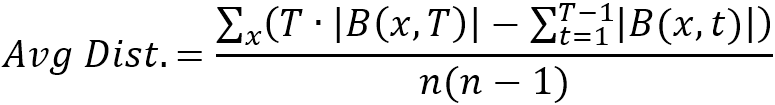where `T` is the maximum shortest distance between nodes in the graph, that is, the diameter of the graph; `n` is the number of nodes in the graph.

### HyperLogLog

HyperLogLog is a cardinality estimation method that counts the cardinality of a sequence after reading its elements. The size of the memory it occupies when computing is a near-linear double logarithm `O(n·log(log n))`, hence the name.

HyperLogLog works as below:

1. Preparing an array `M` of length 2b (array elements are initially set to `-∞`).
2. Mapping each element in the sequence into a sufficiently long binary numeric sequence, use the integer value of the first `b` bits in the binary sequence as the subscript that indicates the position in array.
3. Mapping each element in the sequence into a sufficiently long binary numeric sequence, use the integer value of the first `b` bits in the binary sequence as the subscript that indicates the position in array.
4. After reading all elements, cardinality of the sequence is calculated by the value of the array `M`: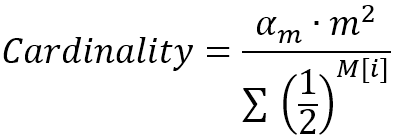where `m` = 2b is the length of array `M`, `α` is the function of `m`: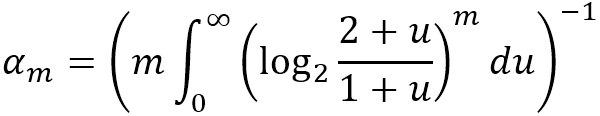For instance, when giving `b` the value of `4`, `m` is `16`, the calculated cardinality is `45.0127``45`.

Ultipa's HyperANF algorithm prepares an array `M` for each node `x` in the graph to calculate `|B(x,t)|`, the number of nodes in the neighborhood set of node `x`, `n` `M` arrays are needed for `n` nodes; define `C` as the `n × m`-dimensional matrix of these arrays: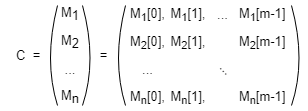Starting from `t` = `1`, calculate `C(t)` iteratively; each Mx in `C(t)` is the maximum element taken from the same subscript from multiple My in `C(t-1)` (`y` is all 1-step neighbors of `x`). Looping iteratively until `C(t)` no longer changes or the number of iterations reaches the limit.

## Special Case

### Lonely Node, Disconnected Graph

Lonely node is not connected with any other node, it does not participate in the calculation of graph average distance.

Connected node pairs are found in each connected component in disconnected graph, graph average distance is calculated by these node pairs jointly.

### Self-loop Edge

Self-loop edge does not form the shortest paths between nodes, thus has no impact on the calculation of graph average distance.

### Directed Edge

For directed edges, HyperANF algorithm ignores the direction of edges but calculates them as undirected edges.

## Results and Statistics

Take the graph below as an example, run the HyperANF algorithm in the graph, set to run 5 iterations the maximum, and the length of array `M` is 24, i.e. `b` = 4: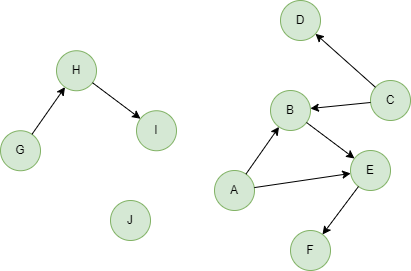Algorithm results: N/A

Algorithm statistics: The estimated graph average distance `hyperANF_result`

hyperANF_result
1.8471822653636683

Note: The accurate graph average distance of this graph is `33/18 = 1.833333`.

## Command and Configuration

• Command: `algo(hyperANF)`
• Configurations for the parameter `params()`:
Name
Type
Default
Specification
Description
loop_num int / >=1, mandatory The maximum number of iterations
register_num int / [4,30], mandatory The value of the power operation exponent `b` which decides the length of array `M` when using HyperLogLog to estimate the cardinality

## Algorithm Execution

#### 1. File Writeback

Not supported by this algorithm.

#### 2. Property Writeback

Not supported by this algorithm.

#### 3. Statistics Writeback

Not supported by this algorithm.

### Direct Return

Alias Ordinal
Type
Description
Column Name
0 KV Graph average distance `hyperANF_result`

Example: Calculate graph average distance, set to run 5 iterations the maximum, and the length of array `M` is 24, i.e. `b` = 4, define algorithm statistics as alias named distance, and return the statistics

``````algo(hyperANF).params({
loop_num: 5,
register_num: 4
}) as distance
return distance
``````

### Streaming Return

Not supported by this algorithm.

### Real-time Statistics

Alias Ordinal
Type
Description
Column Name
0 KV Graph average distance `hyperANF_result`

Example: Calculate graph average distance, set to run 5 iterations the maximum, and the length of array `M` is 24, i.e. `b` = 4, define algorithm statistics as alias named distance, and return the statistics

``````algo(hyperANF).params({
loop_num: 5,
register_num: 4
}).stats() as distance
return distance
``````